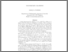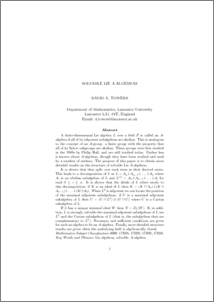# Solvable Lie A-algebras.

Towers, David A. (2011) Solvable Lie A-algebras. Journal of Algebra, 340 (1). pp. 1-12. ISSN 0021-8693Preview
PDF (Solvable_Lie_A-algebras.pdf)
Solvable_Lie_A-algebras.pdf

A finite-dimensional Lie algebra $L$ over a field $F$ is called an $A$-algebra if all of its nilpotent subalgebras are abelian. This is analogous to the concept of an $A$-group: a finite group with the property that all of its Sylow subgroups are abelian. These groups were first studied in the 1940s by Philip Hall, and are still studied today. Rather less is known about $A$-algebras, though they have been studied and used by a number of authors. The purpose of this paper is to obtain more detailed results on the structure of solvable Lie $A$-algebras. \par It is shown that they split over each term in their derived series. This leads to a decomposition of $L$ as $L = A_{n} \dot{+} A_{n-1} \dot{+} \ldots \dot{+} A_0$ where $A_i$ is an abelian subalgebra of $L$ and $L^{(i)} = A_{n} \dot{+} A_{n-1} \dot{+} \ldots \dot{+} A_{i}$ for each $0 \leq i \leq n$. It is shown that the ideals of $L$ relate nicely to this decomposition: if $K$ is an ideal of $L$ then $K = (K \cap A_n) \dot{+} (K \cap A_{n-1}) \dot{+} \ldots \dot{+} (K \cap A_0)$. When $L^2$ is nilpotent we can locate the position of the maximal nilpotent subalgebras: if $U$ is a maximal nilpotent subalgebra of $L$ then $U = (U \cap L^2) \oplus (U \cap C)$ where $C$ is a Cartan subalgebra of $L$. \par If $L$ has a unique minimal ideal $W$ then $N = Z_L(W)$. If, in addition, $L$ is strongly solvable the maximal nilpotent subalgebras of $L$ are $L^2$ and the Cartan subalgebras of $L$ (that is, the subalgebras that are complementary to $L^2$.) Necessary and sufficient conditions are given for such an algebra to be an $A$-algebra. Finally, more detailed structure results are given when the underlying field is algebraically closed.View Item